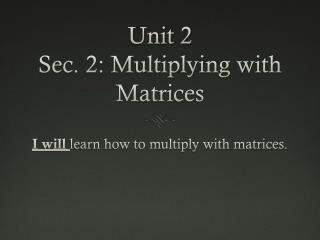DownloadDownload PresentationUnit 2 Sec. 2: Multiplying with Matrices

# Unit 2 Sec. 2: Multiplying with Matrices

Download Presentation## Unit 2 Sec. 2: Multiplying with Matrices

- - - - - - - - - - - - - - - - - - - - - - - - - - - E N D - - - - - - - - - - - - - - - - - - - - - - - - - - -
##### Presentation Transcript

1. Unit 2Sec. 2: Multiplying with Matrices I will learn how to multiply with matrices.

2. INTRO TO MATRICES Matrices A and B can be multiply if and only if the number of ________________ in A is equal to the number of _____________ in B. _____________ ____________ ____________

3. Determine whether each matrix product is defined. If so, state the dimensions of the product. Let , , and CAB

4. Example 1: Find the product , if possible. Let BC = CB =

5. The table shows the scoring summary for LeBron James from the 2009-2013. • His total baskets can be summarized in the baskets matrix B. The point values for each type of basket made can be organized in the point’s value matrix. P. • Write both matrix B and Matrix P. • What matrix operation would compute the total points LeBron James scored for each season? • Which season did LeBron James scored the most points and how many points did he score that season?

6. Assignment Pg. 183-184 #1-11 odd #12-14, #34